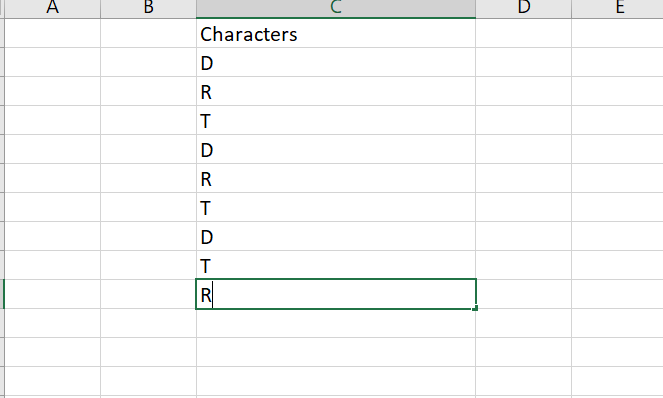# How to get the count of cell values occurrence in column of excel

Hi, I have values in column in excel. I need to find the total number of occurrences of each cell value.
For example:Here, there are different characters in each cell. I want the total count of each character that is Count of D is 3, T is 3, R is 3.

Can you try this,

Thanks!

@Rohit_Patil1

First build a datatble with two columns one is for group a or b or c and another column is for count

`DtClone = (From d In DtBuild.AsEnumerable() Group d By k=d("columnname").toString.Trim Into grp = Group Let coun = grp.count.ToString Let ra = New Object(){k,coun} Select r = DtClone.Rows.Add(ra)).CopyToDataTable()`

DtBuild is the input

DtClone is the putput with two columns.give the column type for both as string

Cheers

Alternately, To Store the Character and it’s Occurence in the form of a Dictionary, we can use the below Expression :

``````characterCount = DT.AsEnumerable.GroupBy(Function(x)x("Characters").ToString).ToDictionary(Function(x)x.First.Item("Characters").ToString,Function(y)y.Count)
``````

Here, `characterCount` is a variable of type Dictionary(Of String,Integer) and `DT` is the input datatable.

Let us know if this doesn’t work.

Hi Rohit

To find the number of cell in column you can use the expression where count is a int32 type variable.

Count= InputDt.AsEnumerable.Select(Function(r) r(“ColumnName”)).ToArray.Count

1 Like

Thank you @Umadevi_Sanjeevi For the response. Will try with this one.

1 Like

Thank you @supermanPunch For the response. Will try with this one.

Thank you @supermanPunch For the response. Let me try with this

1 Like